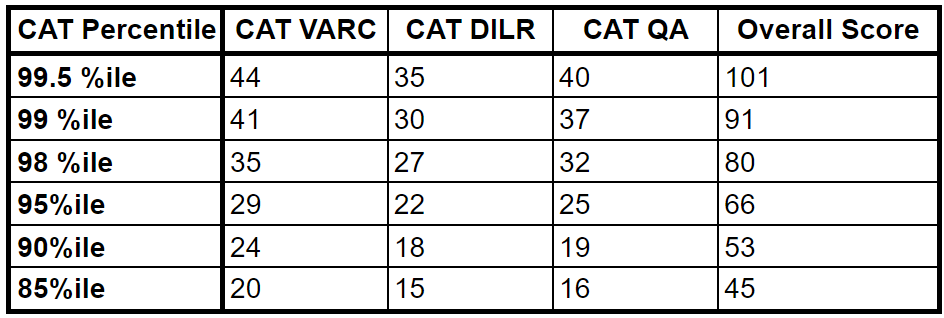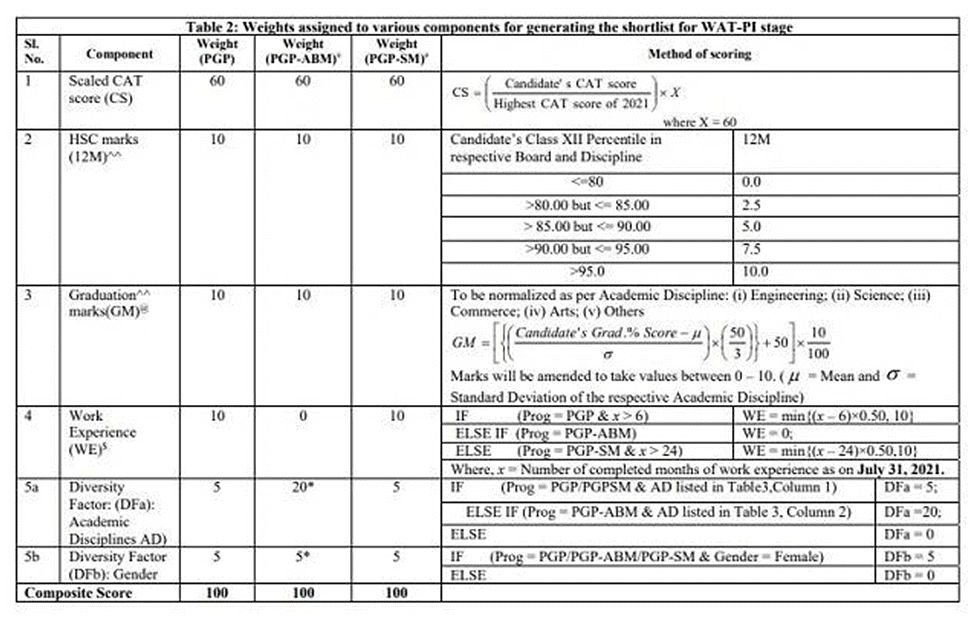CAT  >  CAT Score vs Percentile

# CAT Score vs Percentile

 Table of contentsCAT Score vs PercentileWhat is CAT Percentile?What is CAT Scaled Score?CAT Score vs Percentile 2022Key Changes in CAT Percentile Calculation ProcessWhat is the Normalisation of CAT score?How is the Overall CAT Percentile Calculated?CAT Composite Score CalculationHow to prepare for CAT using EduRev Infinity?Frequently Asked Questions(FAQs) on CAT Score vs Percentile1 Crore+ students have signed up on EduRev. Have you?

CAT aspirants frequently inquire about the distinction between CAT score and CAT percentile, as well as which of the two is more important. Both CAT score and CAT percentile are crucial, as they directly influence a candidate's chances of admission to top B-schools such as IIMs. CAT score is the total marks obtained by a candidate in the exam, whereas CAT percentile is a relative score that compares a candidate's performance to that of other candidates who took the exam.

CAT Score vs Percentile
CAT percentile is highly significant in the screening process for the first round of IIM admission, which includes the Written Ability Test and Personal Interview (WAT-PI). In essence, the CAT percentile measures a candidate's relative score, categorising them based on their performance compared to other candidates. This score is determined by analysing a candidate's marks in the CAT exam along with a few other parameters. CAT results are announced in both score and percentile forms, and most B-schools consider the overall percentile for MBA admissions. To clarify the CAT score vs percentile conundrum, it's essential to comprehend how to calculate the CAT score and CAT percentile.
Before understanding the CAT score, it is important to comprehend the CAT marking scheme.

• The CAT exam consists of 66 questions divided into three sections: Quantitative Ability (24), Verbal Ability and Reading Comprehension (22), and Logical Reasoning and Data Interpretation (20).
• The total marks of the CAT exam are 198, with each correct answer carrying three marks and each incorrect answer carrying a deduction of one mark.
• There is no negative marking for unanswered questions and non-MCQs.
• The CAT score of a candidate is determined by the total score obtained in the exam, which is the sum of marks obtained in each section.
• The sectional scores are added together and sorted to arrive at the total CAT score.

What is CAT Percentile?
CAT percentile is a measure of a candidate's performance in the exam, which represents the percentage of candidates who scored less than or equal to the candidate in question. For instance, a CAT percentile of 99 indicates that the candidate outperformed 99% of the total CAT test takers for that year. In other words, a candidate scoring above 90 percentile would be among the top 10% of students for that year.
If you achieve the top rank in an exam taken by 1,000 people, it means that 999 candidates scored lower than you. As a result, your CAT percentile will be 99.9%, indicating that you performed better than 99.9% of the test takers.
In other words, the CAT percentile does not reflect a candidate's actual performance on the exam but instead measures their performance relative to all the other test takers.

What is CAT Scaled Score?
The CAT exam is conducted in multiple sessions, typically two or three. Each session features question papers that are slightly different from each other. To ensure fairness and equity in comparing the performance of candidates across different sessions, a normalisation process is carried out to calculate a scaled score. The scaled score may be higher or lower than the raw score, as it takes into account the relative difficulty level of the question papers in different sessions.

CAT Score vs Percentile 2022

• In CAT 2022, the percentile has changed for the same CAT score in different exam slots.
• The difficulty level of each exam slot has significantly affected the percentile calculation in CAT 2022.
• Ambiguous questions in the DILR section from CAT slot-2 and slot-3 were not evaluated.
• Due to a decrease in the total maximum score for some slots, a lower CAT score has resulted in a higher percentile.
• Specifically, in CAT slot-2 and slot-3, fewer total marks were available (195 instead of 198) due to one un-evaluated question, which affected the percentile calculation.Key Changes in CAT Percentile Calculation Process

IIM Bangalore has decided to maintain the same criteria for calculating CAT percentiles as in the previous year:

• Percentile scores equal to or above 99.995 will be rounded up to the nearest hundred.
• Scores between 99.985 and 99.995 will be rounded to 99.99, with further rounding for lower percentile scores.

What is the Normalisation of CAT score?

CAT normalisation process is based on GATE scoring, where the normalisation is based on the assumption that distribution of abilities of candidates is the same across all the sessions. The calculations are based on statistic equations.

GATE normalisation takes into account all the variations in the difficulty levels of the question papers across different session.

How is the Overall CAT Percentile Calculated?
A candidate's CAT percentile is calculated based on their scaled score in each of the three sections as well as their overall scaled score. The candidate's CAT scorecard will include their section-wise and overall scaled scores as well as percentiles, which are determined by considering all six measurements.To convert your Raw CAT score to a final percentile, the process involves three stages:

• Raw Score in CAT: You can calculate your raw score based on the marking scheme and the level of accuracy with which you answered the questions. Answer keys made available by IIMs after the exam can also help to verify your raw score.
• Raw Score to Scaled Score: The IIMs will normalize the raw scores for all CAT exam slots and convert them into scaled scores.
• CAT Scaled Score to Percentile Score: The IIMs will then calculate the final percentile score based on the number of test takers across all CAT exam slots.

It is important to understand that while the CAT percentile is a widely used term that represents a candidate's performance relative to other test takers, it is the scaled CAT score that is utilized to calculate the composite score for shortlisting candidates for the WAT-PI round of the IIMs.

CAT Composite Score Calculation
The composite score, which determines admission to programs offered by IIMs, takes into account various factors besides just the CAT score. As a result, two candidates with the same on-paper score may have different composite scores, as other parameters are also considered in the calculation.
To calculate the composite score of a candidate in CAT 2022, certain parameters will be taken into account.

• CAT score (scaled)
• Class 12 marks
• Class 10 marks
• Work Experience
• Diversity (Gender)

How to prepare for CAT using EduRev Infinity?

EduRev Infinity Package for CAT includes:

Also Read other important EduRev Articles given below:

 All about CAT CAT Score vs Percentile CAT Notification CAT Preparation CAT Eligibility Criteria CAT Registration CAT Exam Pattern CAT Study Material CAT Syllabus CAT Previous Year Analysis CAT Sample Papers CAT Mock Tests CAT Previous Year Papers E-Library

Frequently Asked Questions(FAQs) on CAT Score vs Percentile

What is the difference between the CAT score and the CAT percentile?

CAT score is the total marks obtained by a student in the paper while CAT percentile is the candidate’s rank among all the test takers. For admission CAT percentile is taken into consideration.

What is a good score in CAT?

For IIMs, nothing less than 97-98 percentile is good but for other top MBA colleges 90-95 percentile is good. A majority of CAT test takers fall in the category of 80-90 percentile and 100s of B-schools accept candidates having CAT score in this range.

Is scoring 90 percentile in the CAT easy?

90 percentile is not difficult to score in CAT. A large number of students start scoring 90 percentile in mock tests by the time CAT exam nears. However, the journey from 90 to 99 percentile is tough.

How much should I score in CAT to get the 99 percentile?

To aim 99+ percentile in CAT, one should attempt at least 75 per cent questions accurately. Which means they need to solve 2/3rd of total questions in each section. This is achievable with a good strategy of smart selection of questions and time management.

How is the CAT score calculated?

The CAT score is calculated by adding the marks obtained in each section. The marking scheme assigns three marks for every correct answer, and there is a negative marking of one mark for every incorrect answer.

How is the CAT percentile calculated?

The CAT percentile is calculated based on the total number of test-takers, the number of candidates who scored less than the candidate, and the relative performance of candidates in the slot.

Is a high score guaranteed to result in a high percentile?

No, a high score does not guarantee a high percentile as the percentile depends on the performance of other candidates in the exam.

The document CAT Score vs Percentile is a part of CAT category.
All you need of CAT at this link: CAT

### How to Prepare for CAT

Read our guide to prepare for CAT which is created by Toppers & the best Teachers

Track your progress, build streaks, highlight & save important lessons and more!(Scan QR code)

,

,

,

,

,

,

,

,

,

,

,

,

,

,

,

,

,

,

,

,

,

;